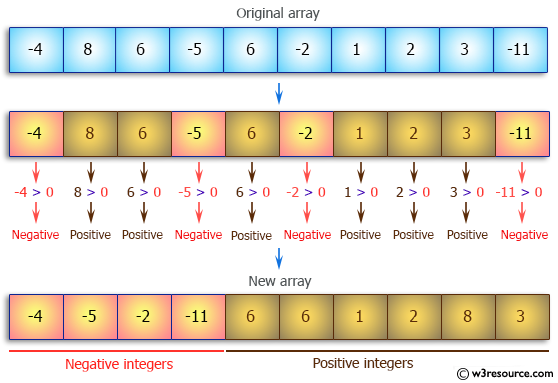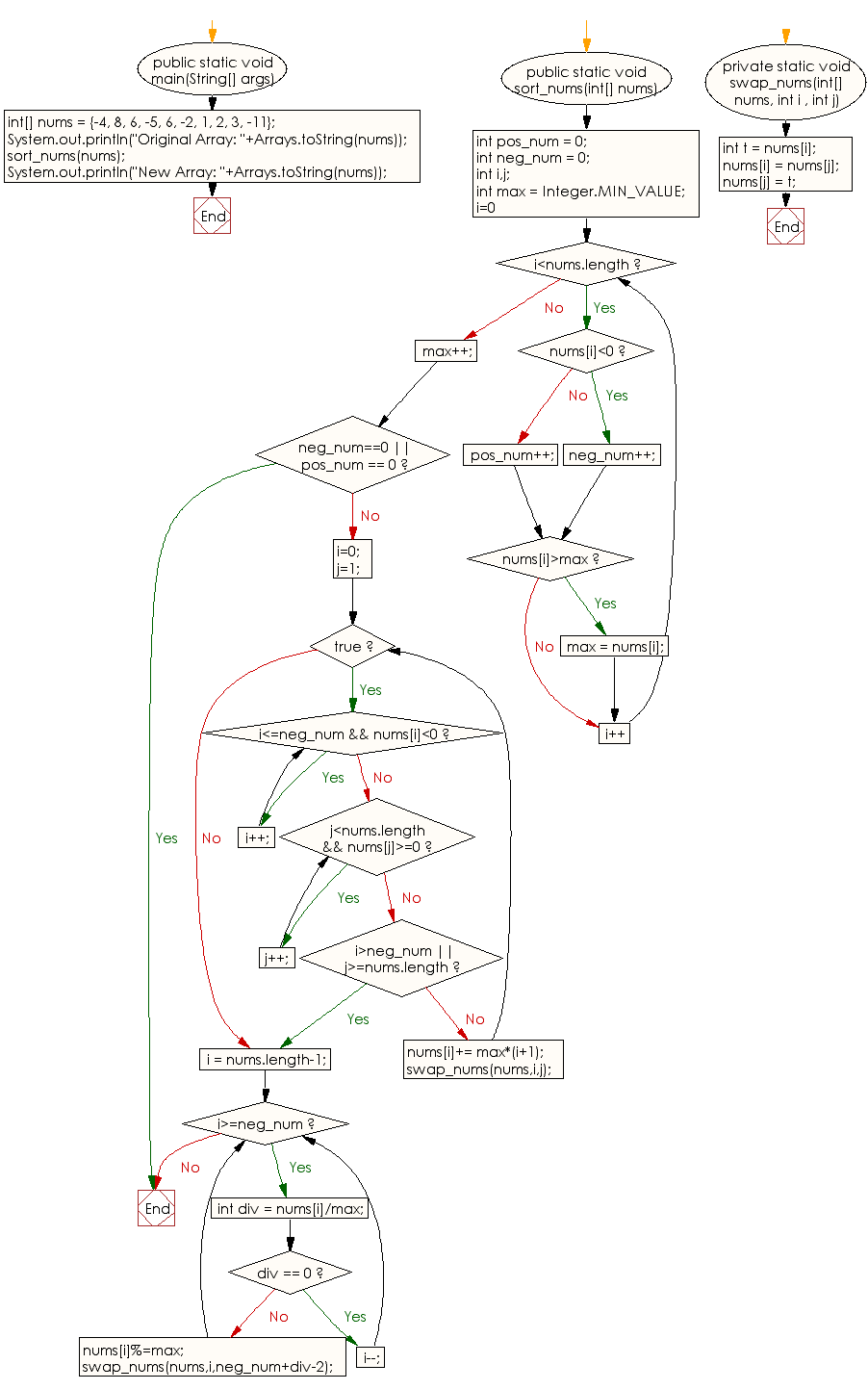﻿ Java: Sort negatives elements before positives in an array# Java Array Exercises: Arrange the elements of a given array of integers where all negative integers appear before all the positive integers

## Java Array: Exercise-48 with Solution

Write a Java program to arrange the elements of a given array of integers where all negative integers appear before all the positive integers.

Pictorial Presentation:Sample Solution:

Java Code:

``````import java.util.Arrays;

public class Main {

public static void main(String[] args) {
int[] nums = {-4, 8, 6, -5, 6, -2, 1, 2, 3, -11};
System.out.println("Original Array: "+Arrays.toString(nums));
sort_nums(nums);
System.out.println("New Array: "+Arrays.toString(nums));
}
public static void sort_nums(int[] nums){
int pos_num = 0;
int neg_num = 0;
int i,j;
int max = Integer.MIN_VALUE;
for(i=0; i<nums.length; i++){
if(nums[i]<0) neg_num++;
else pos_num++;
if(nums[i]>max) max = nums[i];
}
max++;
if(neg_num==0 || pos_num == 0) return;
i=0;
j=1;
while(true){
while(i<=neg_num && nums[i]<0) i++;
while(j<nums.length && nums[j]>=0) j++;
if(i>neg_num || j>=nums.length) break;
nums[i]+= max*(i+1);
swap_nums(nums,i,j);
}

i = nums.length-1;
while(i>=neg_num){
int div = nums[i]/max;
if(div == 0) i--;
else{
nums[i]%=max;
swap_nums(nums,i,neg_num+div-2);
}
}

}
private static void swap_nums(int[] nums, int i , int j){
int t = nums[i];
nums[i] = nums[j];
nums[j] = t;
}
}
```
```

Sample Output:

```
Original Array: [-4, 8, 6, -5, 6, -2, 1, 2, 3, -11]
New Array: [-4, -5, -2, -11, 6, 6, 1, 2, 8, 3]
```

Flowchart:Visualize Java code execution (Python Tutor):

Java Code Editor:

Improve this sample solution and post your code through Disqus

What is the difficulty level of this exercise?

Test your Programming skills with w3resource's quiz.

﻿

## Java: Tips of the Day

countOccurrences

Counts the occurrences of a value in an array.

Use Arrays.stream().filter().count() to count total number of values that equals the specified value.

```public static long countOccurrences(int[] numbers, int value) {
return Arrays.stream(numbers)
.filter(number -> number == value)
.count();
}
```

Ref: https://bit.ly/3kCAgLb